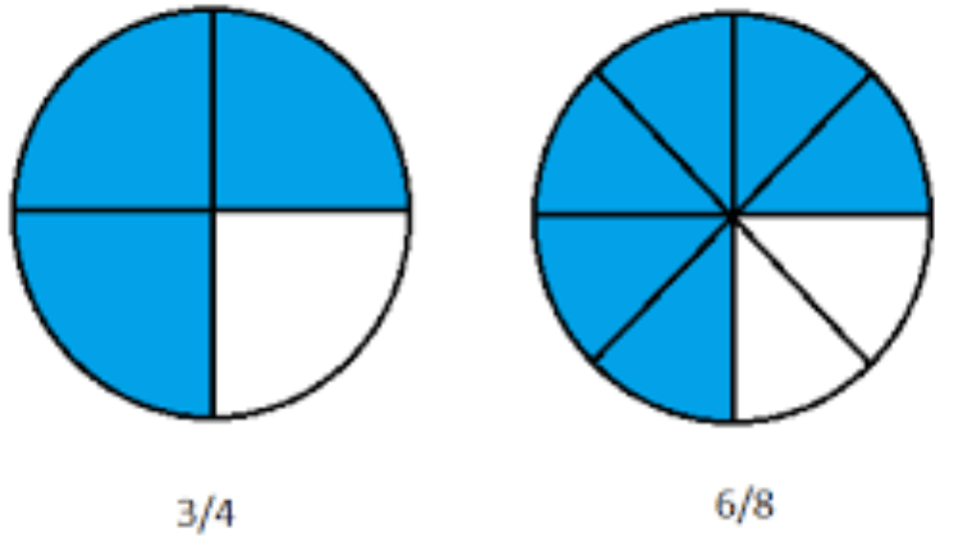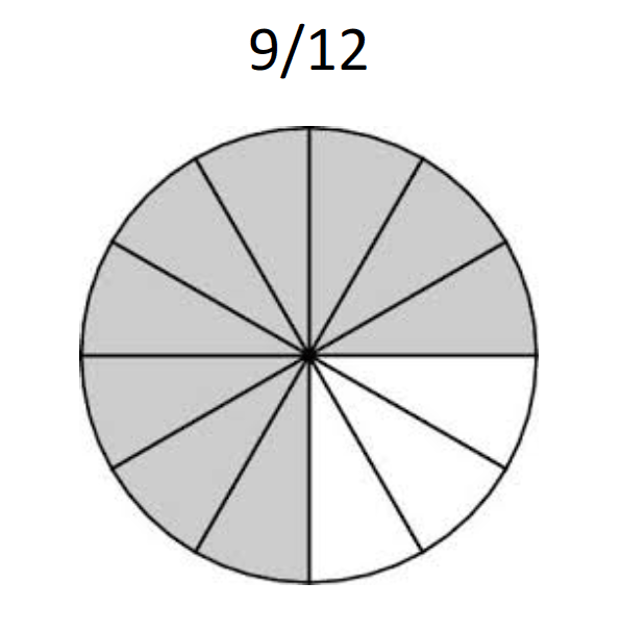## Help with Fractions

Many elementary math students struggle with fractions.  This overview written by one of our math tutors can help students better understand the concept of fractions.

A fraction is used to represent parts of a whole. In order to do this, we use a ratio of two numbers like 3/4.  We call the top number (3) the numerator and the bottom number (4) is called the denominator.  Starting from one whole the denominator tells us how many equal pieces to divide the whole into.  The numerator then tells us how many of those equal pieces we have.  We can visualize 3/4 by taking a whole circle, dividing it into 4 equal pieces, and then filling in 3 of those 4 pieces:### Equivalent Fractions

An important factor in understanding fractions and ratios is that no fraction is unique. Every fraction has infinite equivalent fractions. Take 3/4 and 6/8 as an example:If we continue to divide the circle into more pieces but still fill in the same amount, we will continue to find more and more equivalent fractions.  If we split each of our 4 pieces into 3 we get 12 total pieces with 9 of them filled in.  This means that 9/12 is another fraction that is equivalent to both 3/4 and 6/8.In order to find equivalent fractions numerically, we simply need to multiply or divide the numerator and denominator by the same number. You can use any number you want. If you started with 3/4 and multiplied both the numerator and denominator by 50, you would get the equivalent fraction 150/200. What defines a fraction is the ratio between the numerator and denominator, rather than the actual numbers used.

Understanding equivalent fractions allows us to compare fractions, and to add and subtract fractions with different denominators.

Example: Which is bigger: 3/4 or 7/12?We can use circle models to see that 3/4 uses a larger portion of the circle.

We could also use our knowledge of equivalent fractions to compare them numerically:  3/4 is equal to 9/12.  9/12 is bigger than 7/12, because it takes up two more pieces. Therefore, 3/4 is bigger than 7/12.

Example: What is 2/5 + 4/7?

In order to add these two fractions together we need to put them in the same terms.  We need to find two fractions which are equivalent to ⅖ and 4/7 but with the same denominator.  The easiest way to accomplish this is to find the Least Common Multiple of your two different denominators (any common multiple will work, but the LCM is generally quickest). In this case the Least Common Multiple of 5 and 7 is 35.

Start with 2/5 and then multiply the top and bottom by 7 to get 14/35. Now take 4/7 and multiply the top and bottom by 5 to get 20/35. So ⅖ + 4/7 is equivalent to 14/35 + 20/35.

Now that we have expressed both fractions in terms of 35, we simply add the numerators (or number of pieces) to get 34/35.